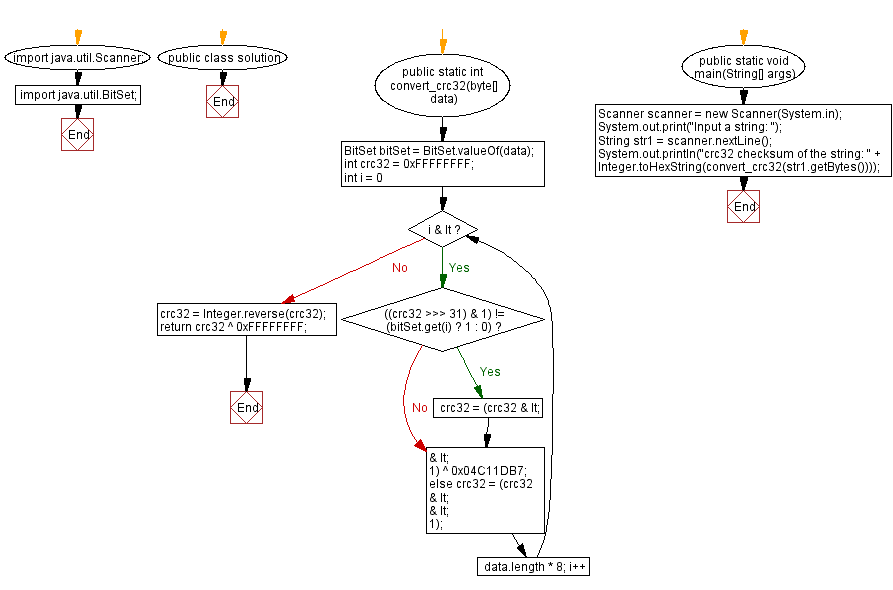# Java: Generate a crc32 checksum of a given string or byte array

## Java Basic: Exercise-206 with Solution

From Wikipedia - A cyclic redundancy check (CRC) is an error-detecting code commonly used in digital networks and storage devices to detect accidental changes to raw data. Blocks of data entering these systems get a short check value attached, based on the remainder of a polynomial division of their contents. On retrieval, the calculation is repeated and, in the event the check values do not match, corrective action can be taken against data corruption. CRCs can be used for error correction.
Write a Java program to generate a CRC32 checksum of a given string or byte array.

Sample Solution:

Java Code:

`````` // Importing required classes from the java.util package
import java.util.Scanner;
import java.util.BitSet;

// Defining a class named "solution"
public class solution {

// Method to convert a byte array to CRC32 checksum
public static int convert_crc32(byte[] data) {
// Creating a BitSet to represent the bits of the input byte array
BitSet bitSet = BitSet.valueOf(data);

// Initializing CRC32 to 0xFFFFFFFF
int crc32 = 0xFFFFFFFF;

// Looping through each bit in the BitSet
for (int i = 0; i < data.length * 8; i++) {
// Checking if the MSB of CRC32 and the current bit in BitSet are different
if (((crc32 >>> 31) & 1) != (bitSet.get(i) ? 1 : 0))
// If different, performing XOR with the polynomial 0x04C11DB7
crc32 = (crc32 << 1) ^ 0x04C11DB7;
else
// If same, shifting CRC32 to the left
crc32 = (crc32 << 1);
}

// Reversing the bits of CRC32
crc32 = Integer.reverse(crc32);

// Returning the final CRC32 checksum by performing XOR with 0xFFFFFFFF
return crc32 ^ 0xFFFFFFFF;
}

// Main method, the entry point of the program
public static void main(String[] args) {
// Creating a Scanner object for user input
Scanner scanner = new Scanner(System.in);

// Prompting the user to input a string
System.out.print("Input a string: ");

// Reading the input string from the user
String str1 = scanner.nextLine();

// Calling the convert_crc32 method and printing the CRC32 checksum in hexadecimal format
System.out.println("crc32 checksum of the string: " + Integer.toHexString(convert_crc32(str1.getBytes())));
}
}
```
```

Sample Output:

```Input a string:  The quick brown fox
crc32 checksum of the string: b74574de
```

Flowchart:Java Code Editor: